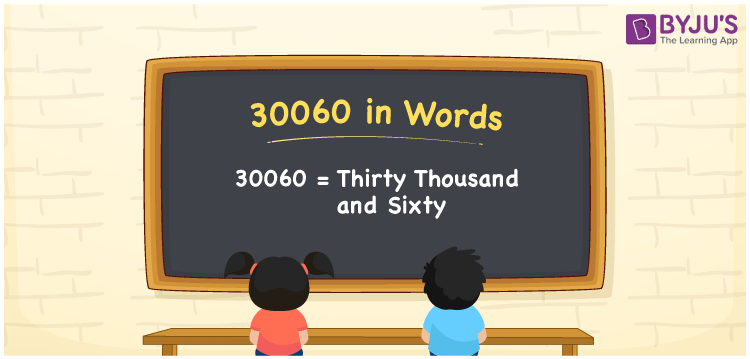# 30060 in Words

30060 in words is Thirty Thousand and Sixty. For example, if you saved Rs. 30060 in a month, you can write, “I have saved Rs. Thirty Thousand and Sixty in a month”. In general, the number name of any number can be written using the ones, tens, hundreds, and thousands place of a number. Thus, the place value chart is useful for writing the number 30060 in words.

 30060 in words Thirty Thousand and Sixty Thirty Thousand and Sixty in Numbers 30060

## 30060 in English Words## How to Write 30060 in Words?

We can convert 30060 to words using a place value chart. This can be done as follows. The number 30060 has 5 digits, so let’s make a chart that shows the place value up to 5 digits.

 Ten thousands Thousands Hundreds Tens Ones 3 0 0 6 0

Thus, we can write the expanded form as:

3 × Ten thousand + 0 × Thousand + 0 × Hundred + 6 × Ten + 0 × One

= 3 × 10000 + 0 × 1000 + 0 × 100 + 6 × 10 + 0 × 1

= 30060

= Thirty Thousand and Sixty

30060 is the natural number that is succeeded by 30061 and preceded by 30059.

30060 in words – Thirty Thousand and Sixty

Is 30060 an odd number? – No.

Is 30060 an even number? – Yes

Is 30060 a perfect square number? – No

Is 30060 a perfect cube number? – No

Is 30060 a prime number? – No

Is 30060 a composite number? – Yes

## Frequently Asked Questions on 30060 in Words

Q1

### How to write 30060 in words?

30060 in English is written as “Thirty Thousand and Sixty”.
Q2

### Is the number 30060 an odd number?

No, the number 30060 is an odd number.
Q3

### Is 30060 a composite number?

Yes, 30060 is a composite number.